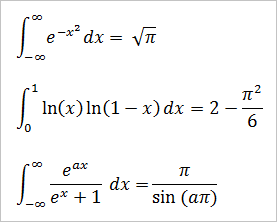# Printable Table of Integrals

The Second Fundamental Theorem of Calculus states that the area under the curve f(x) over the interval [a,b] is equal to F(b) - F(a), where the function F(x) is the antiderivative of f(x). That is, F'(x) = f(x)

You can compute the exact area under a curve or between curves if you know the antiderivative formulas. The process of finding an antiderivative is called integration.

The PDF below is a table of integrals with 180 antiderivative formulas. The formulas fall into seven categories: basic, rational, square root, logarithmic, exponential, trigonometric, and hyperbolic (hyperbolic trig). Click the thumbnail to open the PDF. Calculus formulas are in the public domain, so feel free to print and share.

There are many functions that do not have closed form antiderivatives. For instance, you cannot express the integrals

∫e-x2 dx,   ∫e1/x dx,   ∫√ln(x) dx,   ∫√x³ + 1 dx,   ∫(x + ex)-1 dx,   ∫ln(ex + 1) dx

in terms of elementary functions, e.g., polynomials, roots, exponents, logarithms, etc. To evaluate these types of integrals, you can use numerical algorithms to approximate the area under the curve. Graphing calculators and mathematical software can provide extremely accurate estimates of such integrals.

For some non-integrable functions, you can still evaluate definite integrals over particular intervals. Here are some special definite integrals and their exact values: